Next: Determination of Phase-Shifts Up: Scattering Theory Previous: Partial Waves

# Optical Theorem

The differential scattering cross-section,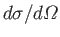, is simply the modulus squared of the scattering amplitude,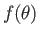. [See Equation (10.28).] The total scattering cross-section is defined as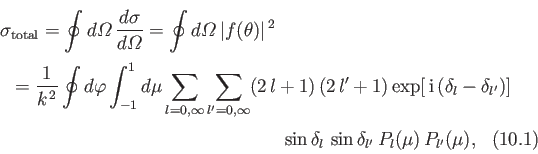where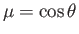. It follows that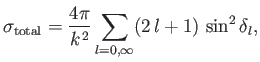(10.89)

where use has been made of Equation (10.65). A comparison of the preceding expression with Equation (10.81) reveals that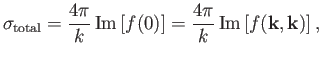(10.90)

because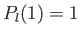. This result is known as the optical theorem [107,73], and is a consequence of the fact that the very existence of scattering requires scattering in the forward (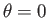) direction, in order to interfere with the incident wave, and thereby reduce the probability current in that direction.

It is conventional to write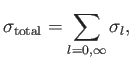(10.91)

where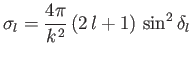(10.92)

is termed theth partial scattering cross-section: that is, the contribution to the total scattering cross-section from theth partial wave. Note that (at fixed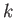) the maximum value for theth partial scattering cross-section occurs when the associated phase-shift,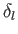, takes the value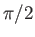.Next: Determination of Phase-Shifts Up: Scattering Theory Previous: Partial Waves
Richard Fitzpatrick 2016-01-22